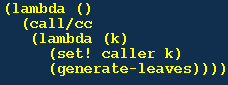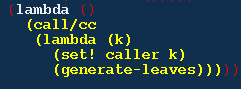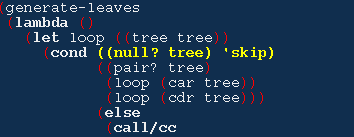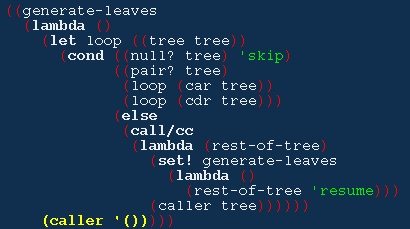# 二叉树匹配问题

## 问题

(1 (2 3)) 和 ((1 2) 3) 是“匹配”的。因为遍历时，我们经过的 叶子依次都是 1, 2, 3.

## 普通办法

```展开 tree1: a, b, c, d, ....
```
```展开 tree2: a, b, d, e, ...
```

## Scheme 的作法

### tree-walker-genenator

```(define tree-walker-genenator
(lambda (tree)
(let ((caller '*))
(letrec
(generate-leaves
(lambda ()
(let loop ((tree tree))
(cond ((null? tree) 'skip)
((pair? tree)
(loop (car tree))
(loop (cdr tree)))
(else
(call/cc
(lambda (rest-of-tree)
(set! generate-leaves
(lambda ()
(rest-of-tree 'resume)))
(caller tree))))))
(caller '()))))
(lambda ()
(call/cc
(lambda (k)
(set! caller k)
(generate-leaves))))))))
```

### tree-match?

```(define tree-match?
(lambda (tree1 tree2)
(let ((walker1 (tree-walker-genenator tree1))
(walker2 (tree-walker-genenator tree2)))
(let loop ()
(let ((leaf1 (walker1))
(leaf2 (walker2)))
(if (eqv? leaf1 leaf2)
(if (null? leaf1) #t (loop))
#f))))))
```

```(define tree1 '(((a b) (y z)) (3 4)))
(define tree2 '(((a b) (t z)) (3 4)))
(define tree3 '(((a (b y) z)) (3 4)))

(tree-match? tree1 tree2)
; #f
(tree-match? tree1 tree3)
; #t
```

## 对于 tree-walker-genenator 函数的详细解释

tree-walker-genenator 的代码有点不好理解。解释一下：

### 细节

• 每次调用 tree-walker-genenator 会生成一个函数，它是最下面 的• 每次调用 tree-walker-genenator 会分配一个局部变量 caller， 它对于函数 walker1，以及本次 define 的环境的其它部分是可见 的，而对于另外的函数，甚至以后再次调用 tree-walker-genenator 生成的新 walker2 都是不可见的。这种 局部空间的用法请参考 LocalVarsAndEnvironments .• 递归的循环过程是由一个新的函数 generate-leaves 进行的。这 个函数是由 letrec 定义的局部函数，它对于 walker1 是可见的， 而对于 walker2 是不可见的。也就是说每个 walker 有自己的 generate-leaves 函数。• 每个 generate-leaves 里面有一个 loop 函数，它就是递归的主 体。如果当前的树是 '() 空表，就返回 'skip；如果当前的树不 是叶子，loop 就对树的两枝分别进行递归调用自己。
• 但是如果当前的树是一个叶子，它就调用 call/cc：• loop 唯一返回的情况，是遇到空表的时候：loop 是在一个 named let 中定义的，它返回就会继续执行 (caller '()) 这个表达式，返回 '() 给 walker1:walker1 得到 '()，从而知道二叉树已经遍历完毕。如果 walker1 再次被调用，它还是会返回到上一次返回 loop 的地方， 然后继续下一次循环，遇到空表，loop 返回 'skip，然后 (caller '())，又得到 '()，以后的调用都会得到 '().

## 另外一种实现

```(define tree-walker-genenator2
(lambda (tree)
(let ((caller '*))
(letrec
((generate-leaves
(lambda (t)
(define orig generate-leaves)
(cond ((null? t) '())
((pair? t)
(generate-leaves (car t))
(generate-leaves (cdr t)))
(else
(call/cc
(lambda (rest-of-tree)
(set! generate-leaves
(lambda (dummy)
(rest-of-tree 'resume)))
(caller t)))
(set! generate-leaves orig))))))
(lambda ()
(call/cc
(lambda (k)
(set! caller k)
(generate-leaves tree))))))))
```

……